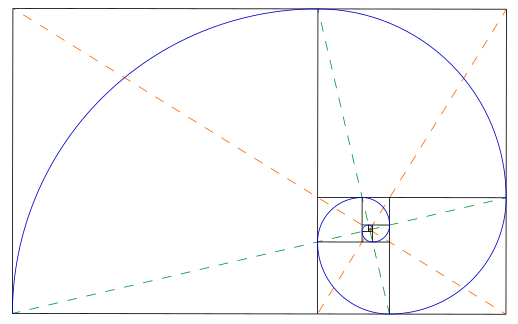# Design FAQ

## Golden Ratio​

• 黄金比例, 黄金分割比
• 常数 - 1.618
• 1:2, 2:3, 3:5, 5:8
• 33:66, 40:60
$\frac {a + b} {a} = \frac a b = \varphi$

a > b > 0

$\varphi = \frac {1 + \sqrt 5} 2 = 1.6180339887498948482...$
$\frac 1 \varphi = \varphi - 1$

$\frac {n + \sqrt {n^2 + 4}} 2$
• n=1 黄金分割 - 1.61803398874989
• n=2 白银分割 - 2.41421356237309
• n=3 青铜分割 - 3.30277563773199## Web 设计 vs 平面 设计​

• 平面设计 - 给人看的
• 在静态的 2D 平面进行设计
• 任何元素都精确定位 - 以拖拉为主
• 不需要考虑客户端环境
• 不存在兼容问题
• 可任意使用本地资源
• 字体、颜色、图片
• 设计可以随心所欲，相同元素之间可以完全不同
• 无交互
• Web 设计 - 给人用的
• 在动态的 2D 环境进行布局
• 元素布局是嵌套在一层一层的流式容器中
• 需要考虑客户端环境
• 设备大小 - 响应式
• 网络带宽 - 资源优化
• 使用人群 - 可访问性 - 残障人群、键盘、鼠标、屏幕、触摸板、屏幕阅读器
• 浏览器 - 系统、字体、版本兼容
• 设计需要考虑元素组件，相同元素组件保持相同 - 便于实现和用户发现功能
• 有交互
• 工业设计 - 产品设计 - 物理层面 - 给人用的